# Python Functions

Working with functions will greatly improve your programming capabilities. You have already worked with a few functions: range(), float(). Now I am going to show you how to build your own.

One great thing about functions is they are reusable. Image you had written a complex formula that calculates final grade based on points earned for 25 tests and assignments. Would you want to have to rewrite that code every time you need it? No, it is much easier just to write it once and call it up when you need it.

Enough talk, let’s build our first function.

## Syntax

def FUNCTION NAME (PARAMETERS):
“””DOCSTRING”””
COMMANDS
RETURN[EXPRESSION]

In the example below, we are not using Return yet. We will get to
that soon enough.

So let’s examine the function below:

• def talkback (strg): = define a new function named talkback which takes one parameter – strg ** note strg is just a variable name I used. It could just as easily been x or y
• “””this prints the string you submit””” = this is the docstring, we will cover this in a minute
• print strg = this is the command portion of the function. This will print the parameter you pass to it.
• ln: talkback(“Print this line”) = this calls the function we had just created. It passes the value “Print this line” to strg, which the function then prints
• help(talkback) — this displays the docstring from the function. Docstrings are instructions that help you to understand how the function works. This is especially important as your functions become more and more complex. Just think of docstrings as help functions.  ** note the triple quotes “”” — this allows you have multiple lines inside the quotes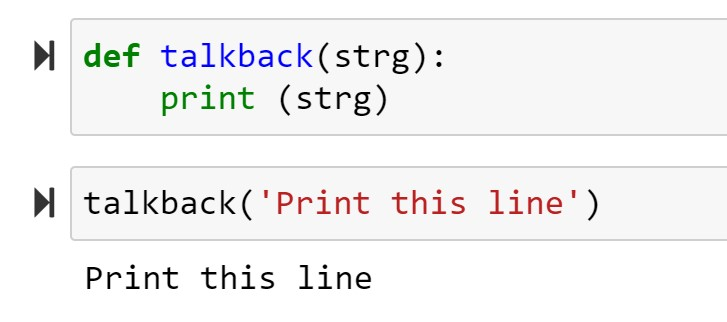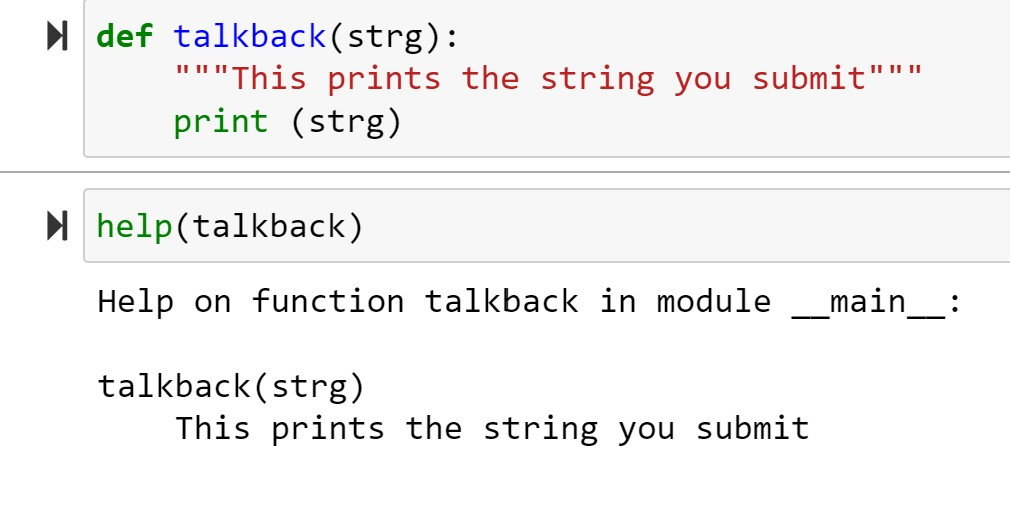Now let’s look at a function using Return

This function, named summed(), is accepting 3 parameters. It then takes the values of the 3 passed parameters and adds them up (assigning them to “s“). Instead of printing the result out, like in the example above, it uses Return s to return the summed value.

The purpose of Return can be seen in ln. As you can see, the summed() function is being used as a number in a division problem. We are able to do this, since the Return command effectively replaces the summed() function with a numeric value in the division equation.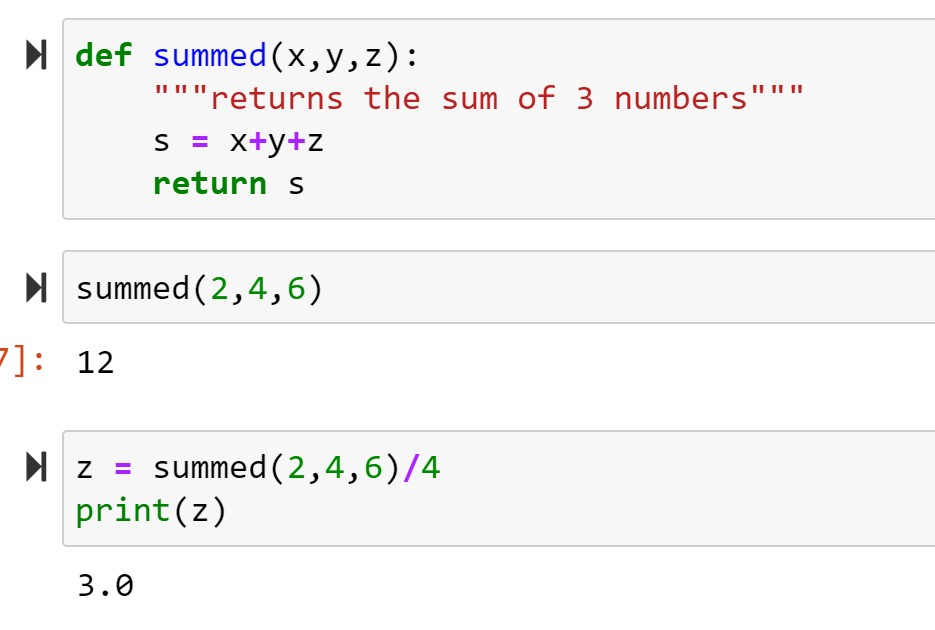Here is what happens if I try using Print instead of Return. Notice the division problem errors out.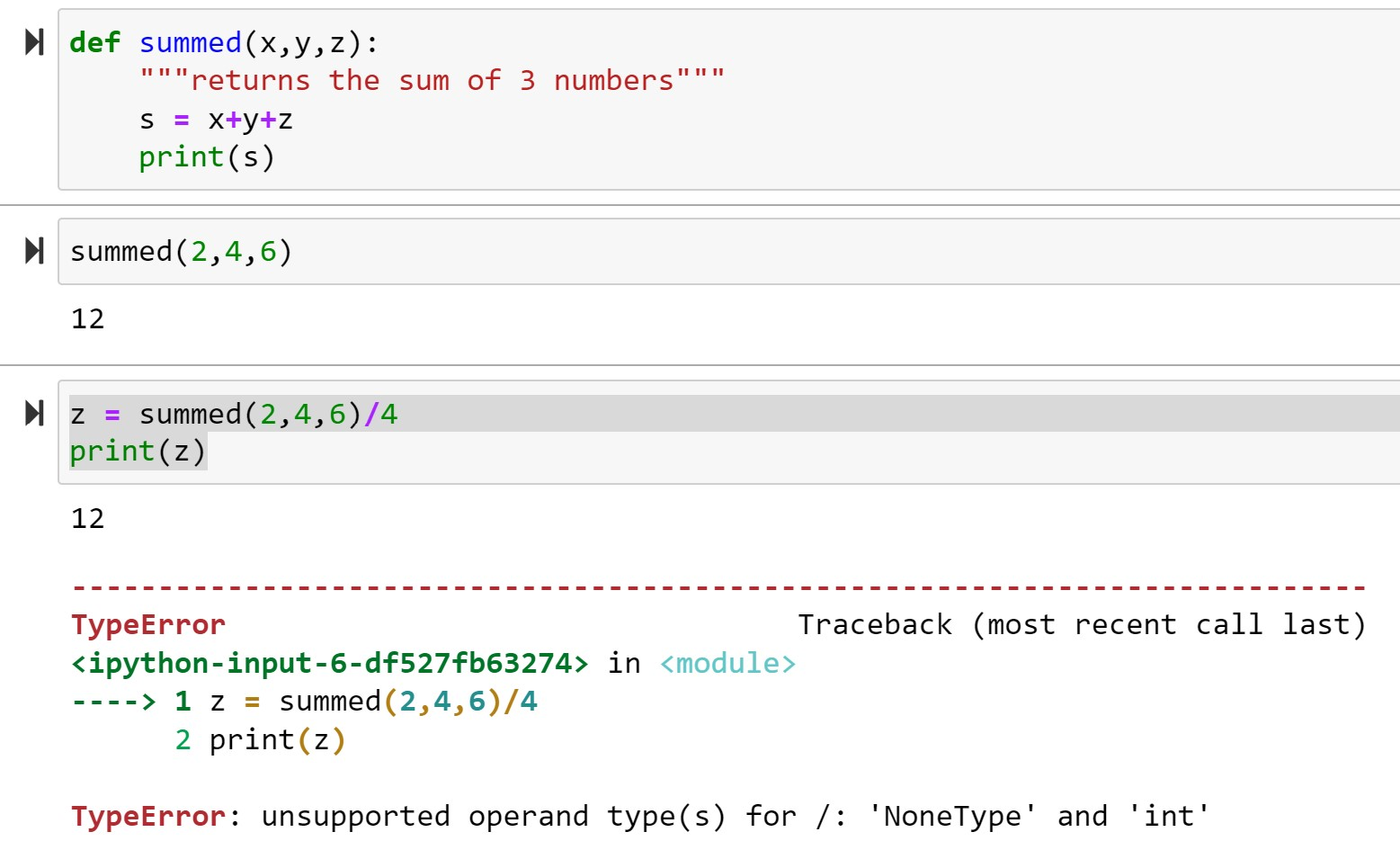Try your code in our online Python console:## Default Arguments and Named Arguments

Let’s look at this function below:

The first thing I want you to notice is the price = 50 in the parameters section. This is called a default argument. As you can see in ln, if I only pass a value for item, function prints out a price of 50 – the default value given.

Now look at ln. When I pass a value for price, it overrides the default value of 50 and sets it to 25

Finally, look at ln. This is naming my arguments. Notice the order of the arguments can be overridden if I pass arguments in the form of parameterName = Value.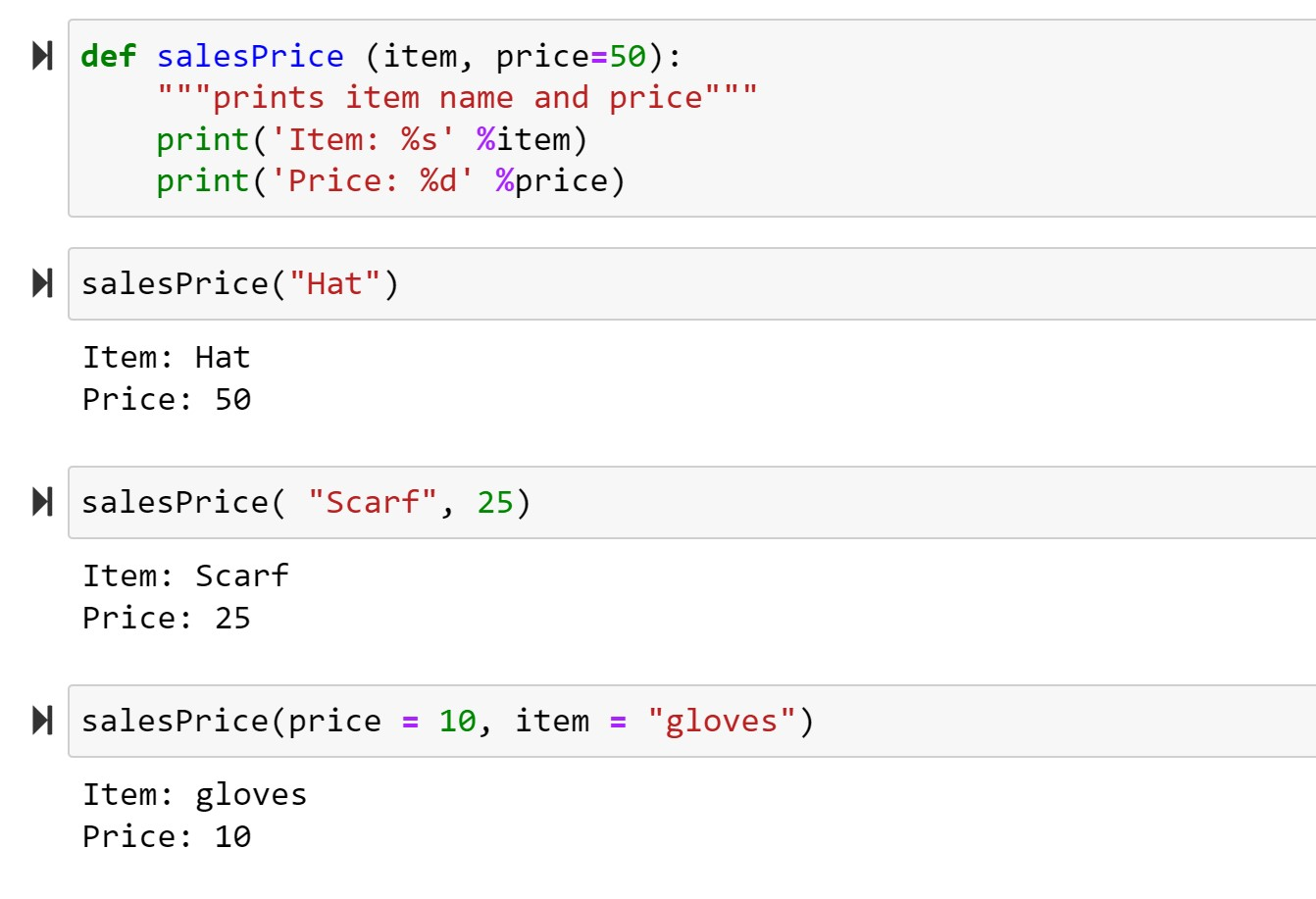Try your code in our online Python console:If you enjoyed this lesson, click LIKE below, or even better, leave me a COMMENT

Previous Lesson: Loops

Next Lesson: Enumerate and Sort

Back to Python Course: Course